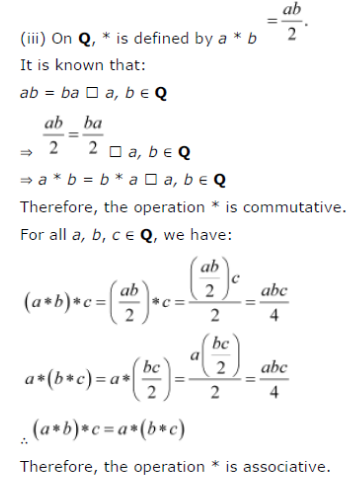# For each binary operation * defined below, determine whether * is commutative or associative:

For each binary operation * defined below, determine whether * is commutative or associative:
(i) On Z, define a * b = a - b
(ii) On Q, define a * b = ab + 1
(iii) On Q, define a * b = ab /2
(iv) On Z, define a * b = 2ab
(v) On Z, define a * b = ab
(vi) On R - {- 1}, define a * b = a /b+1